# 超酷炫！教你如何用 Python 实现动态可视化交互大屏图...随着科技的飞速发展，数据呈现爆发式的增长，任何人都摆脱不了与数据打交道，社会对于 “数据” 方面的人才需求也在不断增大。因此了解当下企业究竟需要招聘什么样的人才？需要什么样的技能？不管是对于在校生，还是对于求职者来说，都显得很有必要。

本文基于这个问题，针对 51job 招聘网站，爬取了全国范围内大数据、数据分析、数据挖掘、机器学习、人工智能等相关岗位的招聘信息。

本次数据分析的特点在于：使用 Tableau 将数据分析的结果做成 可视化交互大屏，效果如下：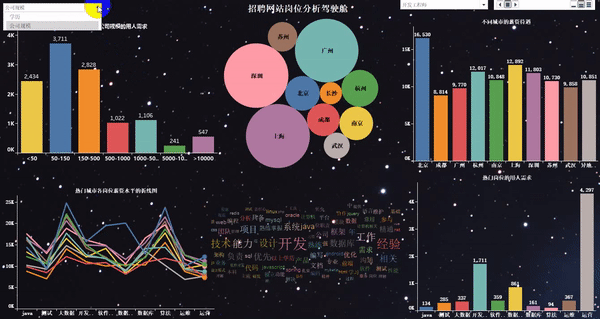1. 爬取岗位：大数据、数据分析、机器学习、人工智能等相关岗位。

2. 爬取字段：公司名、岗位名、工作地址、薪资、发布时间、工作描述、公司类型、员工人数、所属行业。

1. 导入相关库

import requests
import pandas as pd
from pprint import pprint
from lxml import etree
import time
import warnings
warnings.filterwarnings("ignore")



2. 关于翻页的说明

# 第一页的特点
https://search.51job.com/list/000000,000000,0000,00,9,99,%25E6%2595%25B0%25E6%258D%25AE,2,1.html?
# 第二页的特点
https://search.51job.com/list/000000,000000,0000,00,9,99,%25E6%2595%25B0%25E6%258D%25AE,2,2.html?
# 第三页的特点
https://search.51job.com/list/000000,000000,0000,00,9,99,%25E6%2595%25B0%25E6%258D%25AE,2,3.html?



3. 完整的爬取代码

import requests
import pandas as pd
from pprint import pprint
from lxml import etree
import time
import warnings
warnings.filterwarnings("ignore")

for i in range(1,1501):
print("正在爬取第" + str(i) + "页的数据")
url_pre = "https://search.51job.com/list/000000,000000,0000,00,9,99,%25E6%2595%25B0%25E6%258D%25AE,2,"
url_end = ".html?"
url = url_pre + str(i) + url_end
'User-Agent': 'Mozilla/5.0 (Windows NT 10.0; Win64; x64) AppleWebKit/537.36 (KHTML, like Gecko) Chrome/73.0.3683.86 Safari/537.36'
}
web.encoding = "gbk"
dom = etree.HTML(web.text)
# 1、岗位名称
job_name = dom.xpath('//div[@class="dw_table"]/div[@class="el"]//p/span/a[@target="_blank"]/@title')
# 2、公司名称
company_name = dom.xpath('//div[@class="dw_table"]/div[@class="el"]/span[@class="t2"]/a[@target="_blank"]/@title')
# 3、工作地点
# 4、工资
salary_mid = dom.xpath('//div[@class="dw_table"]/div[@class="el"]/span[@class="t4"]')
salary = [i.text for i in salary_mid]
# 5、发布日期
release_time = dom.xpath('//div[@class="dw_table"]/div[@class="el"]/span[@class="t5"]/text()')
# 6、获取二级网址url
deep_url = dom.xpath('//div[@class="dw_table"]/div[@class="el"]//p/span/a[@target="_blank"]/@href')
RandomAll = []
JobDescribe = []
CompanyType = []
CompanySize = []
Industry = []
for i in range(len(deep_url)):
web_test.encoding = "gbk"
dom_test = etree.HTML(web_test.text)
# 7、爬取经验、学历信息，先合在一个字段里面，以后再做数据清洗。命名为random_all
# 8、岗位描述性息
job_describe = dom_test.xpath('//div[@class="tBorderTop_box"]//div[@class="bmsg job_msg inbox"]/p/text()')
# 9、公司类型
company_type = dom_test.xpath('//div[@class="tCompany_sidebar"]//div[@class="com_tag"]/p/@title')
# 10、公司规模(人数)
company_size = dom_test.xpath('//div[@class="tCompany_sidebar"]//div[@class="com_tag"]/p/@title')
# 11、所属行业(公司)
industry = dom_test.xpath('//div[@class="tCompany_sidebar"]//div[@class="com_tag"]/p/@title')
# 将上述信息保存到各自的列表中
RandomAll.append(random_all)
JobDescribe.append(job_describe)
CompanyType.append(company_type)
CompanySize.append(company_size)
Industry.append(industry)
# 为了反爬，设置睡眠时间
time.sleep(1)
# 由于我们需要爬取很多页，为了防止最后一次性保存所有数据出现的错误，因此，我们每获取一夜的数据，就进行一次数据存取。
df = pd.DataFrame()
df["岗位名称"] = job_name
df["公司名称"] = company_name
df["工资"] = salary
df["发布日期"] = release_time
df["经验、学历"] = RandomAll
df["公司类型"] = CompanyType
df["公司规模"] = CompanySize
df["所属行业"] = Industry
df["岗位描述"] = JobDescribe
# 这里在写出过程中，有可能会写入失败，为了解决这个问题，我们使用异常处理。
try:
except:
print("当页数据写入失败")
time.sleep(1)
print("数据爬取完毕，是不是很开心！！！")



在一级页面中，我们爬取了 “岗位名称”，“公司名称”，“工作地点”，“工资”，“发布日期”，“二级网址的 url” 这几个字段。

在二级页面中，我们爬取了 “经验、学历信息”，“岗位描述”，“公司类型”，“公司规模”，“所属行业” 这几个字段。

从爬取到的数据中截取部分做了一个展示，可以看出数据很乱。

1. 相关库的导入及数据的读取

df = pd.read_csv(r"G:\8泰迪\python_project\51_job\job_info1.csv",engine="python",header=None)
# 为数据框指定行索引
df.index = range(len(df))
# 为数据框指定列索引
df.columns = ["岗位名","公司名","工作地点","工资","发布日期","经验与学历","公司类型","公司规模","行业","工作描述"]



2. 数据去重

# 去重之前的记录数
print("去重之前的记录数",df.shape)
# 记录去重
df.drop_duplicates(subset=["公司名","岗位名"],inplace=True)
# 去重之后的记录数
print("去重之后的记录数",df.shape)



3. 岗位名字段的处理

1）岗位名字段的探索

df["岗位名"].value_counts()
df["岗位名"] = df["岗位名"].apply(lambda x:x.lower())



2）构造想要分析的目标岗位，做一个数据筛选

job_info.shape
target_job = ['算法', '开发', '分析', '工程师', '数据', '运营', '运维']
index = [df["岗位名"].str.count(i) for i in target_job]
index = np.array(index).sum(axis=0) > 0
job_info = df[index]
job_info.shape



3）目标岗位标准化处理

job_list = ['数据分析', "数据统计","数据专员",'数据挖掘', '算法',
'大数据','开发工程师', '运营', '软件工程', '前端开发',
'深度学习', 'ai', '数据库', '数据库', '数据产品',
'客服', 'java', '.net', 'andrio', '人工智能', 'c++',
'数据管理',"测试","运维"]
job_list = np.array(job_list)
def rename(x=None,job_list=job_list):
index = [i in x for i in job_list]
if sum(index) > 0:
return job_list[index]
else:
return x
job_info["岗位名"] = job_info["岗位名"].apply(rename)
job_info["岗位名"].value_counts()
# 数据统计、数据专员、数据分析统一归为数据分析
job_info["岗位名"] = job_info["岗位名"].apply(lambda x:re.sub("数据专员","数据分析",x))
job_info["岗位名"] = job_info["岗位名"].apply(lambda x:re.sub("数据统计","数据分析",x))



4. 工资水平字段的处理

job_info["工资"].str[-1].value_counts()
job_info["工资"].str[-3].value_counts()

index1 = job_info["工资"].str[-1].isin(["年","月"])
index2 = job_info["工资"].str[-3].isin(["万","千"])
job_info = job_info[index1 & index2]

def get_money_max_min(x):
try:
if x[-3] == "万":
z = [float(i)*10000 for i in re.findall("[0-9]+\.?[0-9]*",x)]
elif x[-3] == "千":
z = [float(i) * 1000 for i in re.findall("[0-9]+\.?[0-9]*", x)]
if x[-1] == "年":
z = [i/12 for i in z]
return z
except:
return x

salary = job_info["工资"].apply(get_money_max_min)
job_info["最低工资"] = salary.str
job_info["最高工资"] = salary.str
job_info["工资水平"] = job_info[["最低工资","最高工资"]].mean(axis=1)



5. 工作地点字段的处理

#job_info["工作地点"].value_counts()
address_list = ['北京', '上海', '广州', '深圳', '杭州', '苏州', '长沙',
'武汉', '天津', '成都', '西安', '东莞', '合肥', '佛山',
'宁波', '南京', '重庆', '长春', '郑州', '常州', '福州',
'沈阳', '济南', '宁波', '厦门', '贵州', '珠海', '青岛',
'中山', '大连','昆山',"惠州","哈尔滨","昆明","南昌","无锡"]

index = [i in x for i in address_list]
if sum(index) > 0:
else:
return x
job_info["工作地点"] = job_info["工作地点"].apply(rename)



6. 公司类型字段的处理

job_info.loc[job_info["公司类型"].apply(lambda x:len(x)<6),"公司类型"] = np.nan
job_info["公司类型"] = job_info["公司类型"].str[2:-2]



7. 行业字段的处理

# job_info["行业"].value_counts()
job_info["行业"] = job_info["行业"].apply(lambda x:re.sub(",","/",x))
job_info.loc[job_info["行业"].apply(lambda x:len(x)<6),"行业"] = np.nan
job_info["行业"] = job_info["行业"].str[2:-2].str.split("/").str



8. 经验与学历字段的处理

job_info["学历"] = job_info["经验与学历"].apply(lambda x:re.findall("本科|大专|应届生|在校生|硕士",x))
def func(x):
if len(x) == 0:
return np.nan
elif len(x) == 1 or len(x) == 2:
return x
else:
return x
job_info["学历"] = job_info["学历"].apply(func)



9. 工作描述字段的处理

with open(r"G:\8泰迪\python_project\51_job\stopword.txt","r") as f:
stopword = stopword.split()
stopword = stopword + ["任职","职位"," "]

job_info["工作描述"] = job_info["工作描述"].str[2:-2].apply(lambda x:x.lower()).apply(lambda x:"".join(x))\
.apply(jieba.lcut).apply(lambda x:[i for i in x if i not in stopword])
job_info.loc[job_info["工作描述"].apply(lambda x:len(x) < 6),"工作描述"] = np.nan



10. 公司规模字段的处理

#job_info["公司规模"].value_counts()
def func(x):
if x == "['少于50人']":
return "<50"
elif x == "['50-150人']":
return "50-150"
elif x == "['150-500人']":
return '150-500'
elif x == "['500-1000人']":
return '500-1000'
elif x == "['1000-5000人']":
return '1000-5000'
elif x == "['5000-10000人']":
return '5000-10000'
elif x == "['10000人以上']":
return ">10000"
else:
return np.nan
job_info["公司规模"] = job_info["公司规模"].apply(func)



11. 构造新数据

feature = ["公司名","岗位名","工作地点","工资水平","发布日期","学历","公司类型","公司规模","行业","工作描述"]
final_df = job_info[feature]
final_df.to_excel(r"G:\8泰迪\python_project\51_job\词云图.xlsx",encoding="gbk",index=None)


import numpy as np
import pandas as pd
import re
import jieba
import warnings
warnings.filterwarnings("ignore")

df

def get_word_cloud(data=None, job_name=None):
words = []
describe = data['工作描述'][data['岗位名'] == job_name].str[1:-1]
describe.dropna(inplace=True)
[words.extend(i.split(',')) for i in describe]
words = pd.Series(words)
word_fre = words.value_counts()
return word_fre

zz = ['数据分析', '算法', '大数据','开发工程师', '运营', '软件工程','运维', '数据库','java',"测试"]
for i in zz:
word_fre = get_word_cloud(data=df, job_name='{}'.format(i))
word_fre = word_fre[1:].reset_index()[:100]
word_fre["岗位名"] = pd.Series("{}".format(i),index=range(len(word_fre)))


1. 热门城市的用人需求 TOP10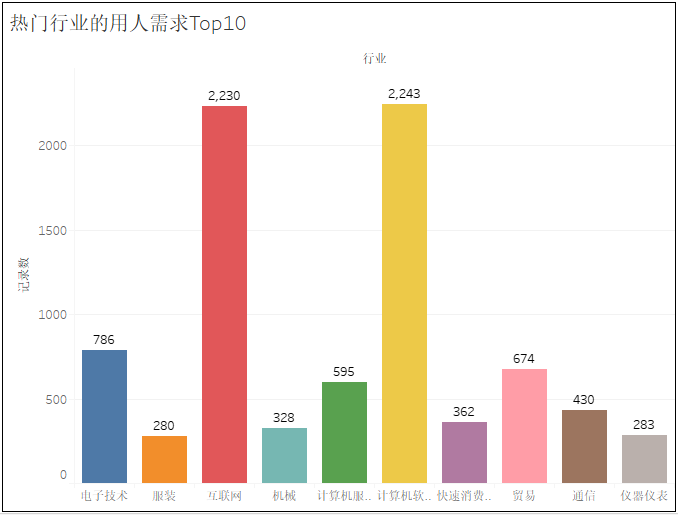2. 热门城市的岗位数量 TOP10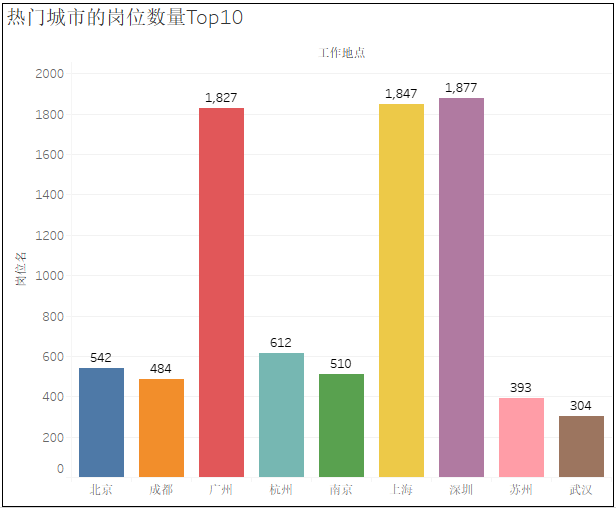3. 不同工作地点岗位数量的气泡图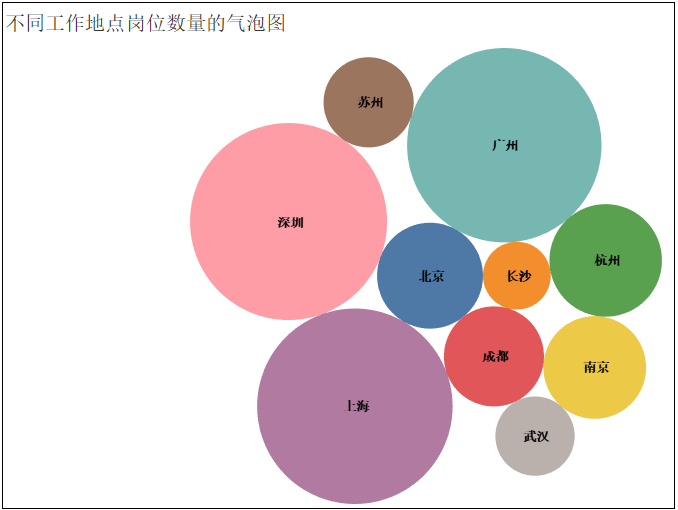4. 热门岗位的薪资待遇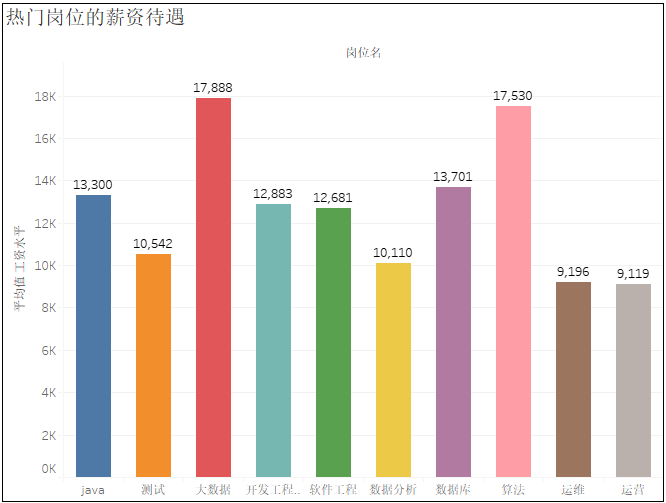5. 热门行业的薪资待遇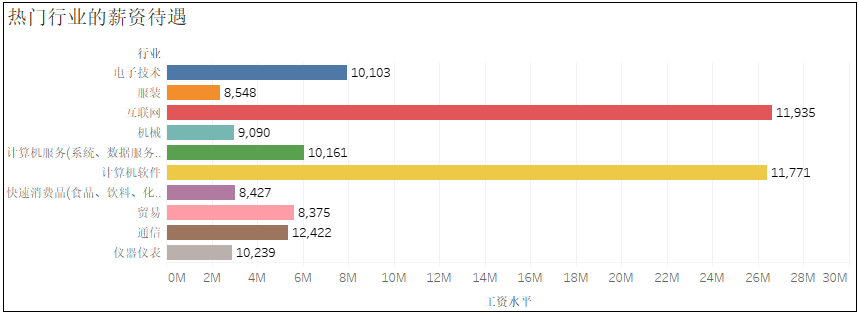6. 可视化大屏的 “动态” 展示太赞了，微软正式推出 Python 零基础教程！

31 个惊艳的数据可视化作品，让你感受 "数据之美"！

GitHub 热榜：歪果小姐姐教你用纯代码画画，真细腻！©️2019 CSDN 皮肤主题: 大白 设计师: CSDN官方博客